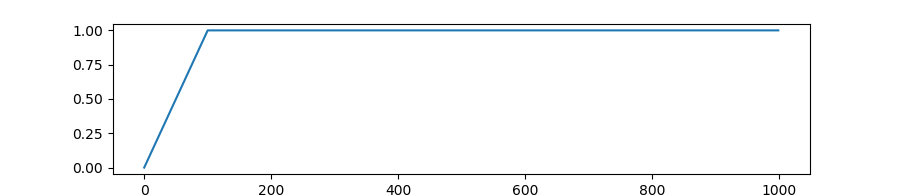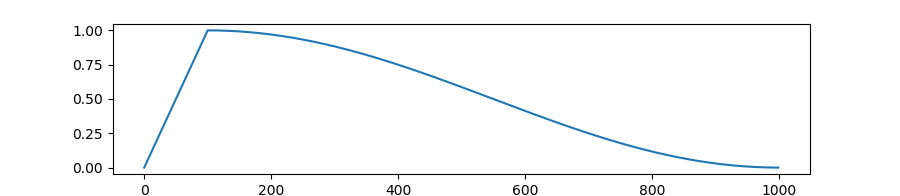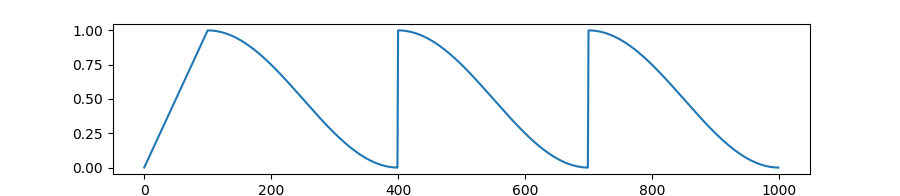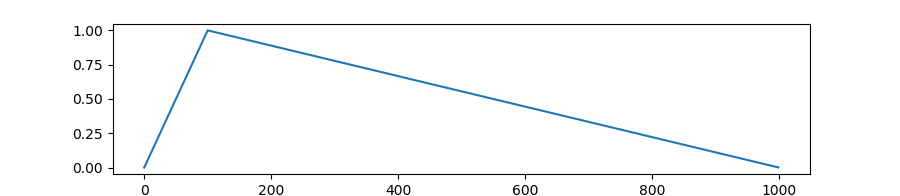# Optimizer¶

The .optimization module provides:

• an optimizer with weight decay fixed that can be used to fine-tuned models, and

• several schedules in the form of schedule objects that inherit from _LRSchedule:

• a gradient accumulation class to accumulate the gradients of multiple batches

## AdamW¶

class transformers.AdamW(params, lr=0.001, betas=0.9, 0.999, eps=1e-06, weight_decay=0.0, correct_bias=True)[source]

Implements Adam algorithm with weight decay fix.

Parameters
• lr (float) – learning rate. Default 1e-3.

• betas (tuple of 2 floats) – Adams beta parameters (b1, b2). Default: (0.9, 0.999)

• eps (float) – Adams epsilon. Default: 1e-6

• weight_decay (float) – Weight decay. Default: 0.0

• correct_bias (bool) – can be set to False to avoid correcting bias in Adam (e.g. like in Bert TF repository). Default True.

step(closure=None)[source]

Performs a single optimization step.

Parameters

closure (callable, optional) – A closure that reevaluates the model and returns the loss.

## AdamWeightDecay¶

class transformers.AdamWeightDecay(learning_rate=0.001, beta_1=0.9, beta_2=0.999, epsilon=1e-07, amsgrad=False, weight_decay_rate=0.0, include_in_weight_decay=None, exclude_from_weight_decay=None, name='AdamWeightDecay', **kwargs)[source]

Adam enables L2 weight decay and clip_by_global_norm on gradients. Just adding the square of the weights to the loss function is not the correct way of using L2 regularization/weight decay with Adam, since that will interact with the m and v parameters in strange ways. Instead we want ot decay the weights in a manner that doesn’t interact with the m/v parameters. This is equivalent to adding the square of the weights to the loss with plain (non-momentum) SGD.

apply_gradients(grads_and_vars, name=None)[source]

This is the second part of minimize(). It returns an Operation that applies gradients.

The method sums gradients from all replicas in the presence of tf.distribute.Strategy by default. You can aggregate gradients yourself by passing experimental_aggregate_gradients=False.

Example:

python grads = tape.gradient(loss, vars) grads = tf.distribute.get_replica_context().all_reduce(‘sum’, grads) # Processing aggregated gradients. optimizer.apply_gradients(zip(grads, vars),

Parameters

• name – Optional name for the returned operation. Default to the name passed to the Optimizer constructor.

• experimental_aggregate_gradients – Whether to sum gradients from different replicas in the presense of tf.distribute.Strategy. If False, it’s user responsibility to aggregate the gradients. Default to True.

Returns

An Operation that applies the specified gradients. The iterations will be automatically increased by 1.

Raises
• TypeError – If grads_and_vars is malformed.

• ValueError – If none of the variables have gradients.

classmethod from_config(config)[source]

Creates an optimizer from its config with WarmUp custom object.

get_config()[source]

Returns the config of the optimizer.

An optimizer config is a Python dictionary (serializable) containing the configuration of an optimizer. The same optimizer can be reinstantiated later (without any saved state) from this configuration.

Returns

Python dictionary.

transformers.create_optimizer(init_lr, num_train_steps, num_warmup_steps, end_lr=0.0, optimizer_type='adamw')[source]

Creates an optimizer with learning rate schedule.

# Schedules¶

## Learning Rate Schedules¶

transformers.get_constant_schedule(optimizer, last_epoch=- 1)[source]

Create a schedule with a constant learning rate.

transformers.get_constant_schedule_with_warmup(optimizer, num_warmup_steps, last_epoch=- 1)[source]

Create a schedule with a constant learning rate preceded by a warmup period during which the learning rate increases linearly between 0 and 1.transformers.get_cosine_schedule_with_warmup(optimizer, num_warmup_steps, num_training_steps, num_cycles=0.5, last_epoch=- 1)[source]

Create a schedule with a learning rate that decreases following the values of the cosine function between 0 and pi * cycles after a warmup period during which it increases linearly between 0 and 1.transformers.get_cosine_with_hard_restarts_schedule_with_warmup(optimizer, num_warmup_steps, num_training_steps, num_cycles=1.0, last_epoch=- 1)[source]

Create a schedule with a learning rate that decreases following the values of the cosine function with several hard restarts, after a warmup period during which it increases linearly between 0 and 1.transformers.get_linear_schedule_with_warmup(optimizer, num_warmup_steps, num_training_steps, last_epoch=- 1)[source]

Create a schedule with a learning rate that decreases linearly after linearly increasing during a warmup period.## Warmup¶

class transformers.WarmUp(initial_learning_rate, decay_schedule_fn, warmup_steps, power=1.0, name=None)[source]

Applies a warmup schedule on a given learning rate decay schedule.

## GradientAccumulator¶
class transformers.GradientAccumulator[source]
Gradient accumulation utility. When used with a distribution strategy, the accumulator should be called in a replica context. Gradients will be accumulated locally on each replica and without synchronization. Users should then call .gradients, scale the gradients if required, and pass the result to apply_gradients`.